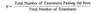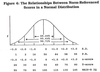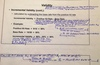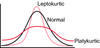# Test Construction Flashcards Preview

## EPPP > Test Construction > Flashcards

Flashcards in Test Construction Deck (71):
1

2

3

## Item Difficulty

### (Ordinal Scale) An item's difficulty level is calculated by dividing the # of individuals who answered the item correctly by the total number of individuals.p=Total # of examinees passing the item/Total # of examinees p value ranges from 0 (nobody answered item correctly; very difﬁcult) to 1.0 (Item answered correctly by all; very easy).An item difficulty index of p=.50 is optimal because it maximizes differentiation between individuals w/high & low ability & helps ensure a high reliability coefficient.Ex: Devel. of EPPP would be interested in assessing Item difficulty level to make sure the exam does not contain too many items that are either too easy or too difficult.1 exception: true/false tests bc probability of answering the question correctly by chance is .50; optimal difficulty level is p=.75 (Item Difficulty Index = p)A multiple choice item w/4 options the probability of answering the item correctly by guessing is 25%; so the optimum p value is halfway btwn 1 & .25, which is 0.625.4

5

6

7

## Item Characteristic Curve (ICC)

### When using IRT, an ICC is constructed for each item by plotting the proportion of examinees in the tryout sample who answered the item correctly against either: The total test score, Performance on an external criterion, or A mathematically-derived estimate of a latent ability or trait. The curve provides info. on the relationship btwn an examinees level on the ability or trait measured by the test & the probability that he/she will respond to the item correctly. The difficulty level of an item is indicated by the ability level (Ex: -3 to +3) where 50% of examinees in sample obtained a correct response. The diff. level for this item is 0 (ave. ability level).The items' ability to discriminate btwn high & low achievers is indicated by the slop of the curve; steeper slope, greater discrimination.Probability of guessing correctly is indicated by the point where the curve intercepts the vertical axis.8

9

10

11

12

13

14

15

16

17

18

19

20

21

22

23

24

25

## Confidence Interval

### Helps a test user to estimate the range w/in which an examinee's true score is likely to fall given their obtained score.Bc tests are not totally reliable, an examinee's obtained score may or may not be his/her true score.Always best to interpret an examinee's obtained score it to construct a confidence interval around that score. A confidence interval indicates the range w/in which an examinee's true score is likely to fall given the obtained score. It is derived using the Standard Error of Measurement (SEM): ​68% = +/- 1 SEM from obtained score 95% = +/- 2 SEM from obtained score 99% = +/- 3 SEM from obtained score ​Standard Error of Measurement (SEM) = Used to construct a confidence interval around a measured or obtained score.26

## Standard Error of Measurement

### It is used to construct a confidence interval around an examinee's obtained (measured) score.Range is calculated by multiplying the standard deviation of the test scores by the square root of 1 - the reliability coefficient.This is an index of the amount of error that can be expected in obtained scores due to the unreliability of the test.SEmes=SDx√(1-rₓₓ)Ex: A psychologist administers an interpersonal assertiveness test to a sales applicant who receives a score of 80. Since test‘s reliability is less than 1.0, the psych. knows that this score might be an imprecise est. of the applicant's true score & decides to use the standard error of measurement to construct a 95% confidence interval. Assuming that the test‘s reliability coefficient is .84 and its standard deviation is 10, the standard error of measurement is equal to 4.0The psych. constructs a 95% conﬁdence interval by adding and subtracting 2 standard errors from the applicant's obtained score: 80 ±2(4.0) = 72 to 88. There is a 95% chance that the applicant's true score falls between 72 and 88.SEM = SDx√(1-rₓₓ)=10√1-.84=10(.4) = 4.027

28

29

30

31

32

33

34

35

36

37

38

39

40

41

42

43

44

45

46

47

48

49

## Incremental Validity

### The extent to which a predictor increases decision-making accuracy when the predictor is used to make selection decisions.Calculated by subtracting the base rate from the positive hit rate.Evaluated by comparing the nymber of correct decisions made with & w/out the new predictor.This has been linked to predictor & criterion cutoff scores; true & false positives; true & false negatives. Scatterplots are used to assess a predictors incremental validity by dividing it into 4 quadrants; predictor cutoff determines if someone is + or -, & criterion determines true or false.50

## True Positive

### Scored high on predictor & criterion;ppl predicted to be successful & are.On scatterplot usually right upper quadrant. (Incremental Validity)51

52

53

## False Negative

### Scored low on predictor & high on criterion;ppl predicted to be unsuccessful but are successful.On scatterplot usually left upper quadrant. (Incremental Validity)54

55

56

57

58

## Norm-Referenced Interpretation

### Involves comparing an examinees test scores to scored obtained in a standardization sample or other comparison group.This type of interpretation may entail converting an examinee's raw score to a percentile rank and/or standard score (e.g., z-scores & T scores). The examinees raw score is converted to a score that indicates his/her relative standing in the comparison group.Percentile Ranks & Standard Scores59

60

61

62

63

64

65

66

67

68

69

70

## Kurtosis

### The degree of peakedness or flatness of a probability distribution, relative to the normal distribution with the same variance. 2 Types: Leptokurtic: Distribution of scores more peaked than a normal distribution. Platokurtic: Distribution of scores flatter than a normal distribution.71